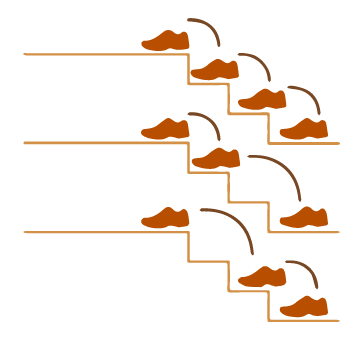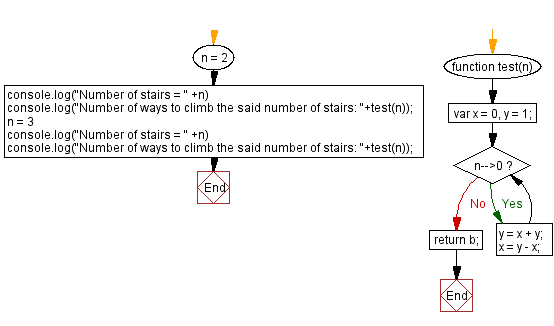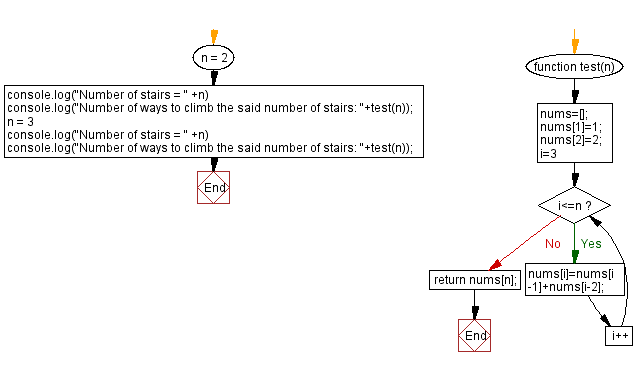# JavaScript: Distinct ways to climb the staircase

## JavaScript Math: Exercise-104 with Solution

A staircase consists of N steps, and you are given the choice of climbing one step at a time or two steps at a time.
Write a JavaScript program to find distinct ways to climb the staircase.

Example:
Number of stairs = 2
There are 2 ways to climb the stairs: (1,1) and (2)
Number of stairs = 3
There are 3 ways to climb the stairs: (1,1,1), (2,1) and (1,2)

Visualisation:Test Data:
(2) -> 2
(3) -> 3

Sample Solution:

HTML Code:

``````<!DOCTYPE html>
<html>
<meta charset="utf-8">
<title>JavaScript program to Distinct ways to climb the staircase</title>
<body>

</body>
</html>
```
```

Solution-1

JavaScript Code:

``````function test(n) {
var x = 0,
y = 1;
while(n-->0){
y = x + y;
x = y - x;
}
return b;
}

n = 2
console.log("Number of stairs = " +n)
console.log("Number of ways to climb the said number of stairs: "+test(n));
n = 3
console.log("Number of stairs = " +n)
console.log("Number of ways to climb the said number of stairs: "+test(n));
```
```

Sample Output:

```Number of stairs = 2
```

Flowchart:Live Demo:

See the Pen javascript-math-exercise-104 by w3resource (@w3resource) on CodePen.

Solution-2

JavaScript Code:

``````function test(n) {
nums=[];
nums=1;
nums=2;
for(i=3;i<=n;i++){
nums[i]=nums[i-1]+nums[i-2];
}
return nums[n];
}

n = 2
console.log("Number of stairs = " +n)
console.log("Number of ways to climb the said number of stairs: "+test(n));
n = 3
console.log("Number of stairs = " +n)
console.log("Number of ways to climb the said number of stairs: "+test(n));
```
```

Sample Output:

```Number of stairs = 2
Number of ways to climb the said number of stairs: 2
Number of stairs = 3
Number of ways to climb the said number of stairs: 3
```

Flowchart:Live Demo:

See the Pen javascript-math-exercise-104-1 by w3resource (@w3resource) on CodePen.

Improve this sample solution and post your code through Disqus

Next: Conversion of integers to English words.

What is the difficulty level of this exercise?

Test your Programming skills with w3resource's quiz.

﻿